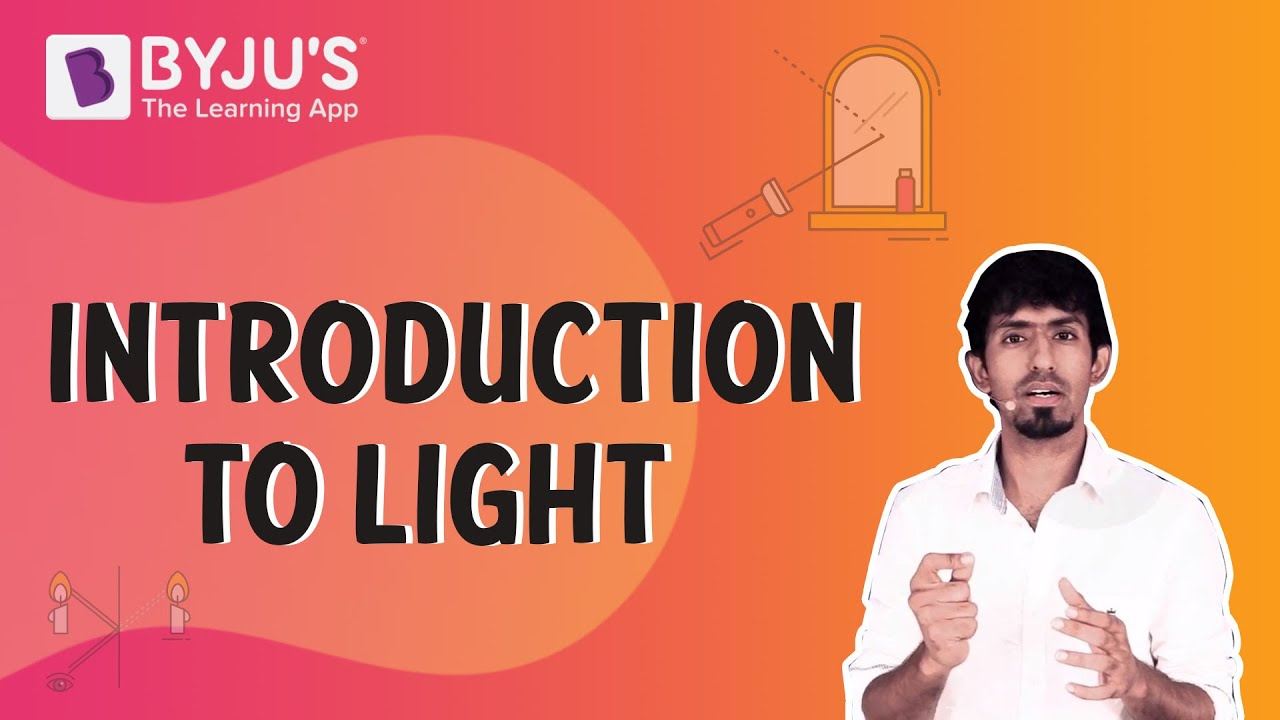# Beer-Lambert Law

The Beer-Lambert law relates the attenuation of light to the material’s properties through which the light travels. Beer-Lambert law is applied to chemical analysis measurements. Let us familiarise ourselves with the law by reading the article.

## What is the Beer-Lambert Law?

Beer’s law states the following:

For a given material, the sample path length and concentration of the sample are directly proportional to the absorbance of the light.

### Various Names for Beer-Lambert Law

The Beer-Lambert law, known by various names such as the Lambert-Beer law, Beer-Lambert–Bouguer law or the Beer’s law. The Beer-Lambert law is known by so many names because more than one law is involved.

• In 1729, Pierre Bouguer discovered the law.
• Later, in 1760, Johann Heinrich Lambert quoted Bouger’s discovery saying that the absorbance of a sample is directly proportional to the path length of light. Although Lambert dint claim the discovery, he was often credited with it.
• In 1852, August Beer discovered a related law which stated that the absorbance is proportional to the concentration of the sample.
 What is Beer’s Law? Beer law states that concentration and absorbance are directly proportional to each other and it was stated by August Beer. What is Lambert Law? Lambert law states that absorbance and path length are directly proportional and it was stated by Johann Heinrich Lambert.

## Beer-Lambert Law Equation

The Beer-Lambert law equation is as follows:

 $$\begin{array}{l}\large I=I_{0}e^{-\mu (x)}\end{array}$$

Where,

I is the intensity

I0 is the initial intensity

μ is the coefficient of absorption

x is the depth in metre

Learn how to derive the Beer-Lambert Law equation by visiting the page below:

### Beer-Lambert Law Applications

This law finds applications in various fields such as:

#### Analytical chemistry

This analysis mainly concentrates on the separation, quantification, and identification of matter by spectrophotometry. There is no involvement of extensive pre-processing of the sample to get the results. For example, bilirubin count in a blood sample can be determined by using a spectrophotometer.

#### In atmosphere

Solar or stellar radiation in the atmosphere can be described using this law. The law in atmospheric applications has a modified equation:

$$\begin{array}{l}\large T=e^{-m(T_{a}+T_{g}+T_{RS}+T_{NO2}+T_{w}+T_{O3}+T_{r}+..)}\end{array}$$

Where,

a is the aerosols

g is the mixed gases

RS is the Raman scattering effect.

NO2 is Nitrogen dioxide

w is the water vapour absorption

O3 is Ozone

r is Rayleigh scattering

### Beer-Lambert Law Limitations

Using this law it becomes easy to study the absorptivity coefficient of the sample when the concentration is low i.e. <10mM but as the concentration becomes high i.e. >10mM there is a deviation as the electrostatic interactions become more.

Related Articles

Does light behave like a wave? or does it behave like a particle? What is darkness? How do we see objects? These are some of the fundamental questions we often ask ourselves. Find answers to these questions by watching the video below.## Frequently Asked Questions – FAQs

### Who discovered the Beer-Lambert law?

Pierre Bouger discovered the law in 1729.

### What does the Beer’s Law state?

Beer’s Law or the Beer-Lambert Law states that the amount of energy absorbed or transmitted by a solution is proportional to the solution’s molar absorptivity and the concentration of solute.

### What is the Beer-Lambert law equation used for?

The Beer-Lambert law relates the attenuation of light to the material’s properties through which the light is travelling. The law is applied to the analysis of a mixture by spectrophotometry without extensive pre-processing of the sample.

### Why is Beer-Lambert law important?

Beer’s law is important in the field of physics, chemistry and meteorology. The law is used in chemistry to measure the concentration of chemical solutions, analyse oxidation, and measure polymer degradation. The law also explains the attenuation of radiation through the Earth’s atmosphere.

### When does the Beer-Lambert law fail?

The law fails at high concentrations (>0.01M) due to electrostatic interactions between molecules in proximity.

Stay tuned to BYJU’S and Fall in Love with Learning!

Test your knowledge on Beer-Lambert law